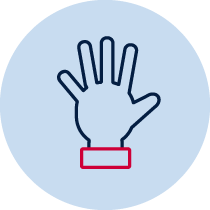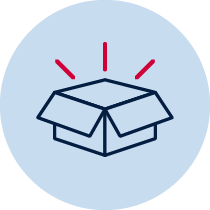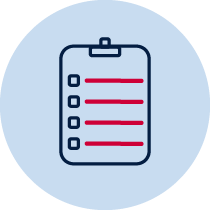# Fractions and decimals – halves and quarters

Check students’ understanding of fraction and decimal concepts.Practical Build and make Resource required Verbal recording Take photo Teacher observationIndividual Whole class Collaborative

## Number and algebra – fractions and decimals

• describes mathematical situations and methods using everyday and some mathematical language, actions, materials, diagrams and symbols MA1-1WM
• represents and models halves, quarters and eighths MA1-7NA

## Content

Recognise and describe one-half as one of two equal parts of a whole (ACMNA016)

## National Numeracy Learning Progression mapping to the NSW mathematics syllabus

When working towards the outcome MA1-7NA the sub-elements (and levels) of Multiplicative strategies (MuS1-MuS2, MuS5) and Interpreting fractions (InF1) describe observable behaviours that can aid teachers in making evidence-based decisions about student development and future learning.

## Materials

• paper squares, rectangles or strips in different sizes e.g. A4, A5 paper, coloured squares
• pencils or a device for recording

## Teacher instructions

This task is designed to check student’s understanding of the fraction and decimal concepts:

• one-half is one of two equal parts of a whole
• modelling half of a whole object
• two equal parts create a whole object,
• the term halves refer to two equal parts of a whole
• fraction notation for half

Students are given some paper rectangles and fold each rectangle to show halves. Discuss whether the parts are equal.

Students label the parts of the rectangle using the words or fraction notation for half. Students then turn the paper over and fold the paper into quarters and label each quarter using the words or fraction notation.

## Student instructions

Brainstorm, with students, the language that we can use for the parts when we fold a piece of paper (half, halves, quarters for example) and write these up on a board or chart for students to use.

Brainstorm, with students, any other ways we could write these words and include these on the board or chart (fraction notations for half and quarter). Tell students that they will be folding a piece of paper into halves and could use the words or notations on the board to label the parts after folding.

• choose a piece of paper and fold it in half any way that they would like
• turn and talk to a partner and tell them how they know their paper is folded in half. This can be recorded on a device for analysis. Ask students and record some of these on another chart for discussion in another lesson.
• label the parts of their paper
• turn the paper over and fold it into half again and label the parts
• talk about their paper folds with a partner and record this on a device.

## Possible areas for further exploration

Students:

• could not fold their paper into equal halves
• could not label their paper using words or the fraction notation for half
• could not label their paper using words or the fraction notation for quarter

## Where to next?

Explicitly teach students the concept of one-half (see ES1 content)

• Explicitly teach the language and symbols for recording fractions using concrete materials, pictures and objects (food, blocks, rods)

Students can repeat the activity using:

• a strip of paper to create a continuous model, linear to compare fractional parts based on length
• columns or bars including chocolate or lamingtons.

Adapted from – Stage 1 fractions and decimals

Early fraction development